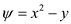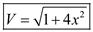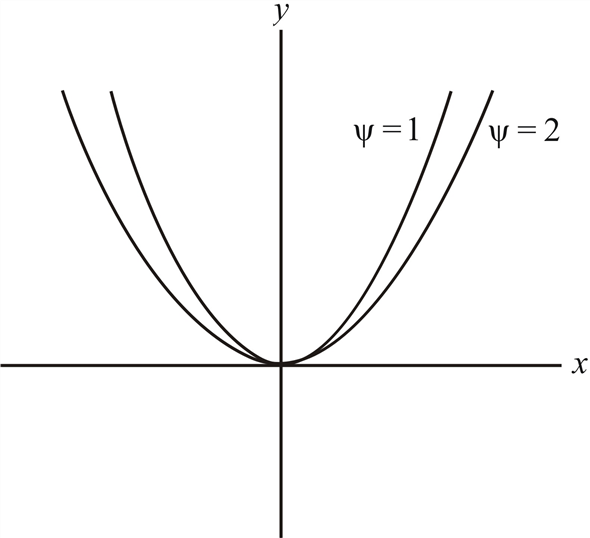# A flow field is described by the equation ψ = y-x² Sketch the streamlines ψ = 0, ψ=1,and ψ = 2. Derive an expression for the velocity V at any point in the flow field.Calculate the vorticity.

Question-AnswerCategory: Fluid MechanicsA flow field is described by the equation ψ = y-x² Sketch the streamlines ψ = 0, ψ=1,and ψ = 2. Derive an expression for the velocity V at any point in the flow field.Calculate the vorticity.

A flow field is described by the equation ψ = y-x² Sketch the streamlines ψ = 0, ψ=1,and ψ = 2. Derive an expression for the velocity V at any point in the flow field.Calculate the vorticity.

Step: 1

The equation for the flow field isFrom the condition for stream function we get the velocity coordinateasSimilarly we get the velocity coordinateasStep: 2

Thus the magnitude of the velocity isTherefore the expression for the velocity at any point in the flow field isStep: 3

The vorticity of the flow is determined by using the relationSince the vorticity of the flow is not equal to zero we can say that the flow is rotational

Step: 4

From the equation for the flow field iswe can say that the flow field is a family of parabolas symmetric about the